# Reliability Growth Planning

 Chapter 4: Reliability Growth Planning

 Chapter 4 Reliability Growth Planning

## Contents

Available Software:
RGA

More Resources:
RGA Examples Collection

## Introduction

In developmental reliability growth testing, the objective is to test a system, find problem failure modes, incorporate corrective actions and therefore increase the reliability of the system. This process is continued for the duration of the test time. If the corrective actions are effective then the system mean time between failures (MTBF) or mean trials between failures (MTrBF) will move from an initial low value to a higher value. Typically, the objective of reliability growth testing is not to just increase the MTBF/MTrBF, but to increase it to a particular value called the goal or requirement. Therefore, determining how much test time is needed for a particular system is generally of particular interest in reliability growth testing.

The Duane postulate is based on empirical observations, and it reflects a learning curve pattern for reliability growth. This learning curve pattern forms the basis of the Crow-AMSAA (NHPP) model. The Duane postulate is also reflected in the Crow Extended model in the form of the discovery function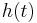$h(t)\,\!$.

The discovery function is the rate in which new, distinct problems are being discovered during reliability growth development testing. The Crow-AMSAA (NHPP) model is a special case of the discovery function. Consider that when a new and distinct failure mode is first seen, the testing is stopped and a corrective action is incorporated before the testing is resumed. In addition, suppose that the corrective action is highly effective that the failure mode is unlikely to be seen again. In this case, the only failures observed during the reliability growth test are the first occurrences of the failure modes. Therefore, if the Crow-AMSAA (NHPP) model and the Duane postulate are accepted as the pattern for a test-fix-test reliability growth testing program, then the form of the Crow-AMSAA (NHPP) model must be the form for the discovery function,$h(t)\,\!$.

To be consistent with the Duane postulate and the Crow-AMSAA (NHPP) model, the discovery function must be of the same form. This form of the discovery function is an important property of the Crow extended model and its application in growth planning. As with the Crow-AMSAA (NHPP) model, this form of the discovery function ties the model directly to real-world data and experiences.

## Growth Planning Models

There are two types of reliability growth planning models available in RGA:

## Growth Planning Inputs

The following parameters are used in both the continuous and discrete reliability growth models.

### Management Strategy Ratio & Initial Failure Intensity

When a system is tested and failure modes are observed, management can make one of two possible decisions, either to fix or to not fix the failure mode. Therefore, the management strategy places failure modes into two categories: A modes and B modes. The A modes are all failure modes such that, when seen during the test, no corrective action will be taken. This accounts for all modes for which management determines to be not economical or otherwise justified to take a corrective action. The B modes are either corrected during the test or the corrective action is delayed to a later time. The management strategy is defined by what portion of the failures will be fixed.

Let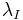${{\lambda }_{I}}\,\!$ be the initial failure intensity of the system in test.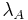${{\lambda }_{A}}\,\!$ is defined as the A mode's initial failure intensity and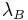${{\lambda }_{B}}\,\!$ is defined as the B mode's initial failure intensity.${{\lambda }_{A}}\,\!$ is the failure intensity of the system that will not be addressed by corrective actions even if a failure mode is seen during testing.${{\lambda }_{B}}\,\!$ is the failure intensity of the system that will be addressed by corrective actions if a failure mode is seen during testing.

Then, the initial failure intensity of the system is:\begin{align} {{\lambda }_{I}}={{\lambda }_{A}}+{{\lambda }_{B}} \end{align}\,\!

The initial system MTBF is: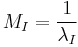${{M}_{I}}=\frac{1}{{{\lambda }_{I}}}\,\!$

Based on the initial failure intensity definitions, the management strategy ratio is defined as: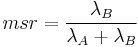$msr=\frac{{{\lambda }_{B}}}{{{\lambda }_{A}}+{{\lambda }_{B}}}\,\!$

The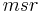$msr\,\!$ is the portion of the initial system failure intensity that will be addressed by corrective actions, if seen during the test.

The failure mode intensities of the type A and type B modes are: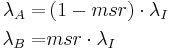\begin{align} {{\lambda }_{A}}= & \left( 1-msr \right)\cdot {{\lambda }_{I}} \\ {{\lambda }_{B}}= & msr\cdot {{\lambda }_{I}} \end{align}\,\!

### Effectiveness Factor

When a delayed corrective action is implemented for a type B failure mode, in other words a BD mode, the failure intensity for that mode is reduced if the corrective action is effective. Once a BD mode is discovered, it is rarely totally eliminated by a corrective action. After a BD mode has been found and fixed, a certain percentage of the failure intensity will be removed, but a certain percentage of the failure intensity will generally remain. The fraction decrease in the BD mode failure intensity due to corrective actions,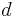$d\,\!$,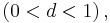$\left( 0 is called the effectiveness factor (EF).

A study on EFs showed that an average EF,$d\,\!$, is about 70%. Therefore, about 30% (i.e.,$100(1-d)%\,\!$) of the BD mode failure intensity will typically remain in the system after all of the corrective actions have been implemented. However, individual EFs for the failure modes may be larger or smaller than the average. This average value of 70% can be used for planning purposes, or if such information is recorded, an average effectiveness factor from a previous reliability growth program can be used.

### MTBF Goal

When putting together a reliability growth plan, a goal MTBF/MTrBF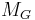${{M}_{G}}\,\!$ (or goal failure intensity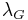${{\lambda }_{G}}\,\!$ ) is defined as the requirement or target for the product at the end of the growth program.

### Growth Potential

The failure intensity remaining in the system at the end of the test will depend on the management strategy given by the classification of the type A and type B failure modes. The engineering effort applied to the corrective actions determines the effectiveness factors. In addition, the failure intensity depends on$h(t)\,\!$, which is the rate at which problem failure modes are being discovered during testing. The rate of discovery drives the opportunity to take corrective actions based on the seen failure modes, and it is an important factor in the overall reliability growth rate. The reliability growth potential is the limiting value of the failure intensity as time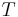$T\,\!$ increases. This limit is the maximum MTBF that can be attained with the current management strategy. The maximum MTBF/MTrBF will be attained when all type B modes have been observed and fixed.

If all the discovered type B modes are corrected by time$T\,\!$, that is, no deferred corrective actions at time$T\,\!$, then the growth potential is the maximum attainable with the type B designation of the failure modes and the corresponding assigned effectiveness factors. This is called the nominal growth potential. In other words, the nominal growth potential is the maximum attainable growth potential assuming corrective actions are implemented for every mode that is planned to be fixed. In reality, some corrective actions might be implemented at a later time due to schedule, budget, engineering, etc.

If some of the discovered type B modes are not corrected at the end of the current test phase, then the prevailing growth potential is below the maximum attainable with the type B designation of the failure modes and the corresponding assigned effectiveness factors.

If all type B failure modes are discovered and corrected with an average effectiveness factor,$d\,\!$, then the maximum reduction in the initial system failure intensity is the growth potential failure intensity: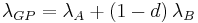${{\lambda }_{GP}}={{\lambda }_{A}}+\left( 1-d \right){{\lambda }_{B}}\,\!$

The growth potential MTBF/MTrBF is:${{M}_{GP}}=\frac{1}{{{\lambda }_{GP}}}\,\!$

Note that based on the equations for the initial failure intensity and the management strategy ratio (given in the Management Strategy and Initial Failure Intensity section), the initial failure intensity is equal to: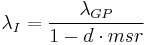${{\lambda }_{I}}=\frac{{{\lambda }_{GP}}}{1-d\cdot msr}\,\!$

### Growth Potential Design Margin

The Growth Potential Design Margin (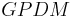$GPDM\,\!$ ) can be considered as a safety margin when setting target MTBF/MTrBF values for the reliability growth plan. It is common for systems to degrade in terms of reliability when a prototype product is going into full manufacturing. This is due to variations in materials, processes, etc. Furthermore, the in-house reliability growth testing usually overestimates the actual product reliability because the field usage conditions may not be perfectly simulated during testing. Typical values for the$GPDM\,\!$ are around 1.2. Higher values yield less risk for the program, but require a more rigorous reliability growth test plan. Lower values imply higher program risk, with less safety margin.

During the planning stage, the growth potential MTBF/MTrBF,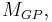${{M}_{GP}},\,\!$ can be calculated based on the goal MTBF,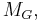${{M}_{G}},\,\!$ and the growth potential design margin,$GPDM\,\!$.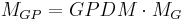${{M}_{GP}}=GPDM\cdot {{M}_{G}}\,\!$

or in terms of failure intensity: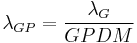${{\lambda }_{GP}}=\frac{{{\lambda }_{G}}}{GPDM}\,\!$